# 153 - Trinity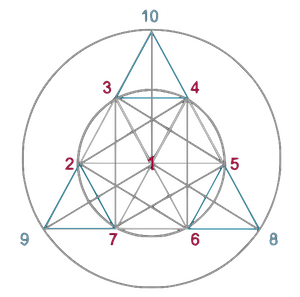1996/97

The 153 is a special number and yet it is hardly recognized by anyone. For people of Central European descent it plays a major role in the Bible: it is the number of universality. Therefore, it is well-known that (risen) Jesus showed the apostles where to fish and that they caught 153 fish without tearing the web (John 21: 5). Some Catholics may know that the Rosary Psalter - these are the prayers with the Rosary - includes 153 "Ave Maria" and 153 small beads on the string of the Rosary. In fact, the meaning of 153 is much broader, and the numerical ratios I would like to show are older than Christianity - sometimes at least 4000-6000 years.

Also the coding is very old and was in particular part of many old languages. In Hebrew, Aramaic or ancient Greek alphabets, the letters are assigned to numerical values. For about 1000 years there is a whole science, called Gematria or Numerology, in various versions, because the alphabets have different quantities of letters. That in turn offers even better corner-codings because of even more room for even more (mis-) interpretations - something like that is probably also desired when codings are incorporated. But there were also codings that should only serve the beauty or exaltation of the text. .

Go to, let us go down, and there confound their language, that they may not understand one another's speech.

Genesis 11:7

With this little essay I want to try to raise a little bit more the awareness of "cultural numbers in our spinal cord" (in the subconscious mind) and to show a whole matrix in which our life is "measured". One compass is the Tetraktys (picture) - more on that later.

## 153 - in Music

Tonic, fifth, third. The "Triad" (1 3 5) in the order of appearance in the series of natural harmonics (1 5 3). These are again the 1st, 3rd and 5th tone of the harmonic series. The musical notation shows the first 5 partials. The two octaves are not counted, because they are repetitions of "the same" tone, i.e. frequency doublings. Nevertheless, it should be mentioned that 3 fundamental tones (111) sound up until the third (3).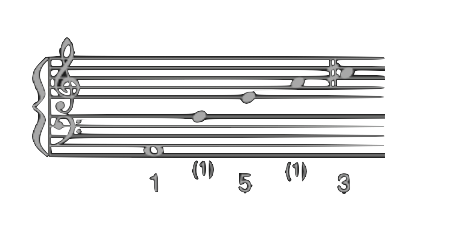Of course, there is much more to this order. It is a major triad and major stands for male, minor for female. As interesting as it is, I leave it to stay on topic. So much to say, this may have more to do with the man's positioning "ahead of the woman" than muscle mass or patriarchal fantasies. The minor sounds build up only on the basis of a major sound. It's about vibrations and frequencies.

In our tonal order, there are 12 (chromatic) tones. To harmonically arrange the chord tones, and to derive the corresponding scale - this is done in thirds (3rd steps) - we need 2 octaves - so 24 (2 x 12) chromatic tones. The notes of the first octave form the chord root (1 3 5) plus seventh (7), the second octave the overtones 9, 11, 13 - which serve as passing notes (2, 4, 6) of the resulting scale. The 2 x 12 chromatic tones are reminiscent of the 2 x 12 hours on the clock. The resulting diatonic "church modes" contain 7 tones and create 7 modes, one week has 7 days, and God created the world in 7 days. 7 stands for perfection.

## Spectral Colors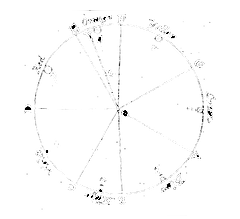It is interesting to compare our scales with the 7 visible spectral colors in Newton's "Color Circle". First, we can see that the colors orange and indigo have a narrower "pie slice" (corresponding to the visible width of each color). It corresponds approximately to a halving. The positions of orange and indigo correspond to the positions of the two contained semitones of a major scale [Ionic]: from the third to the fourth and from the seventh to the octave. If we halve all other major pieces, we get an (almost) equal twelve division of the circle (clock/chromatic scale). Here lies the connection of the musical term "chromatic" ("in colors") to the spectrum.

Newton's "Color Circle" is an interpretation of Newton, who allegedly, by adding indigo, wanted to approximate the ancient Greek Sophists. However, the widths of the visible spectral colors are not a matter of interpretation and the perceived colors relate to the human eye.

The merge of digits: 1 octave has 7 steps and 1 week has 7 days (17).

Back to 153:

## Characteristics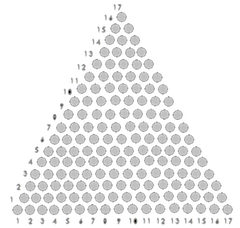153 is the 17th triangular number ( Pythagoras ) - i.e., it results from the addition of the first 17 natural numbers:

1 + 2 + 3 + 4 + 5 + 6 + 7 + 8 + 9 + 10 + 11 + 12 + 13 + 14 + 15 + 16 + 17 = 153.

In the image can be seen why triangular numbers are called triangular: they always form an (equilateral) triangle.

The addition of the base number 17 with 153 leads to the tenfold: 153 + 17 = 170

Zero is meaningless in numerology. This is due to the fact that, at the time of writing, our modern, decimal number system (including 0) was not yet introduced. The spelling of numbers was additive at that time (e.g. XVII).

The cross sum of 153 multiplied by 17 again gives 153, the 17th triangular number.

1 + 5 + 3 = 9 and 9 x 17 = 153. The coefficients in prime numbers: 3 x 3 x 17 = 153.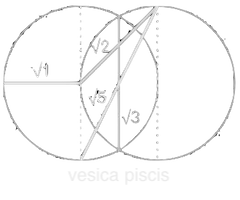The number 153 corresponds to the sum of the cubes (3) of its digits:

13 + 33 = 1 + 125 + 27 = 153
Thus, she is one of the four three-digit narcissistic [Armstrong] numbers.

As a factorial calculation it is: 1! + 2! + 3! + 4! + 5! = 153
1*1 + 1*2 + 1*2*3 + 1*2*3*4 + 1*2*3*4*5 = 1 + 2 + 6 + 24 + 120 = 153

The cross sum of 153 is 32
1 + 5 + 3 = 9 = 3 x 3 = 32

The sum of all dividends of 153 gives (3 x 3)2
1 + 3 + 9 + 17 + 51 = 81 = 92 = (3 x 3)2

The prime numbers contained in the series 1-17 squared add up to 666
22 + 32 + 52 + 72 + 112 + 132 + 172 = 4 + 9 + 25 + 49 + 121 + 169 + 289 = 666

Every number, divisible by 3, will end up at 153 by adding up the cubic numbers of their digit sums and the following ones, as shown in the following examples:

## Two Types

The two examples show the two types of the "trinity algorithm".

While the Type 1 results continuously become smaller (1485, 702, 351, 153), the Type 2 values also become larger in between (9, 729, 1080, 513, 153).

There are 666 instances of Type 2 up to the 2001 value, the end of the algorithm.

There are 6 permutations of 153. In both examples, we look at a permutation of 153 (351 and 513) as the last summation value before 153.

## Type 1

399 is divisible by 3
[399 / 3 = 133]

33 + 93 + 93 =
27 + 729 + 729 = 1485

13 + 43 + 83 + 53 =
1 + 64 + 512 + 125 = 702

7 3 + 03 + 23 =
343 + 0 + 8 = 351

33 + 53 + 13 =
27 + 125 + 1 = 153

13 + 53 + 33 =
1 + 125 + 27 = 153

## Type 2

1200 is divisible by 3
[1200 / 3 = 400]

1200 ist durch 3 teilbar
[1200 / 3 = 400]

13 + 23 + 03 + 03 =
1 + 8 + 0 + 0 = 9

93 = 729

73 + 23 + 93 =
343 + 8 + 729 = 1080

13 + 03 + 83 + 03 =
1 + 0 + 512 + 0 = 513

53 + 13 + 33=
125 + 1 + 27 = 153

13 + 53 + 33 =
1 + 125 + 27 = 153

## 153 and 3π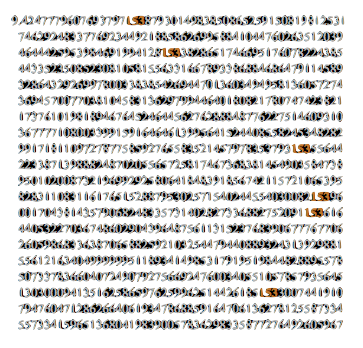The connection of the 153 with 3π (PI) is also interesting. Within the first 1000 digits behind the decimal point, the 153 appears six times. 6 is the digit for weakness, failure and for man. I had chosen 1000 digits, because the numbers up to 1000 are God's numbers and above 1000 those of man. 3π because of the Trinity.

Let's look at the positions in which the 153 appears: After the decimal point it is the 17th, 123rd, 443rd, 596th, 645th and 888th position. Here, the 17th and the 888th catch my eye, that is, the first and last figures, because 888 is the numerical value for "Jesus" (ιησους [ι+η+σ+ο+υ+ς ⇒ 10 + 8 + 200 + 70 + 400 + 200 = 888]) and 17 for "good" (טוב).

The position digits in the addition:
17 + 123 + 443 + 596 + 645 + 888 = 2712.
The cross sum is 12 (2 + 7 + 1 + 2 = 12)

In fact, the Hebrew Bible even has a sentence that corresponds to 2712 (Daniel 7:16):

קרבת על חד מן קאמיא ויציבא אבעא מנה על כל דנה ואמר לי ופשר מליא יהודענני

The words correspond to the values
205 + 81 + 586 + 40 + 247 + 59 + 50 + 100 + 95 + 74 + 119 + 152 + 90 + 12 + 100 + 702 = 2712.

I came near unto one of them that stood by, and asked him the truth of all this. So he told me, and made me know the interpretation of the things.

Daniel 7:16

## 153 in the Bible

The 17th word of the Hebrew Bible is God, the 153rd word is "good" (טוב), which corresponds to the Gematria value of 17. It shouldn't be unmentioned that 17 is the sum of 7, the number of the perfection and work of the Holy Spirit, and 10, the number of rules and laws (10 commandments). As digits there is the 7 behind the 1. The 1 stands for the Creator.

In the Old Testament, the word "preaching" is used 153 times, in the New Testament the words "darkness" and "Peter" appear 153 times each.

The Apocalypse uses 153 numbers greater than 1.

It is reported by 153 blessings through Jesus Christ and Jesus "Kingdom" (ministry) lasted 153 weeks (Sabbaths) - from his 30th to 33rd year of life (333: the divine number).

Many biblical expressions concerning fishing or fish are divisible by 153 (Greek): Fish = 153, the Net or "God's Creation" = 1224 (153 x 8), Fisher of Men = 2142 (153 x 14), the multitude of fishes = 2448 (153 x 16), to cast the net = 3060 (153 x 20).

I find it particularly interesting that there are two bindings in the "multitude of fishes" (= 2448): once for unversality (16 x 153) and once for the unit of measurement (17 x 144) - with 17 (triangular number 153) as multiplier. Also interesting is that "to cast the net" adds to 3060 - without zeros: 36 (triangle 666). Also the circle (360 °) plus 2 x 153 (306) is equal to 666.

Regarding the apostles who caught 153 fish without tearing the net:
The number for "net", 1224 (= 8 x 153), we find as number combination also in the day/night division (12/24 hours), as date on Christmas Eve (12/24) and in the church music: 2 octaves of 12 tones for the creation of the [7-step] diatonic scale - thus 7 out of 12 tones. There were also only 7 of the 12 apostles who caught the 153 fish. And only one (Simon Peter) pulled the net ashore.

In the 153rd verse of the Genesis, a reference is made to the length measure "cubit" (144 cubits = 12 x 12).

In the 153rd verse of the revelation a reference to the calendar is made (5 months = 150 - 153 days).
In the same verse again the length measure (cubit) is mentioned in the description of the wall (12 x 12 = 144).

In various passages of the Bible, 7 months and 153 days are mentioned (e.g. the Flood). 7 months + 153 days = 1 year = 12 months.

The (3 x 12 =) 36th (triangle 666) and final chapter in the Book of Numbers is the 153rd chapter of the Bible.

## A brief summary up to this point:

The cultural imprint of Europe was made by Christianity (born of Judaism), whose content goes back to Babylon via the Mithras cult, and which, from the New Testament, speaks of the Trinity.

We have seen several abnormalities of the universal number 153 - most had to do with the 3. Whether triangular number, triad, divisibility - the 3 plays a prominent role, even as a square or cubic number (32 and 33) - and the 153 itself is three digits.

Abraham, Isaac and Jacob are considered the 3 forefathers of Judaism. Three central biblical "structures" consist of 3 floors: Noah's Ark, Solomon's Temple, and the Second Temple.

Already in the Old Testament, the 3 has a prominent role and the combination to the 333 is evident. (Gen 15: 9) "Bring me a three-year-old young cow, a three-year-old goat and a three-year-old ram ...". (1 Chronicles 21: 9) God to King David: "... three years of famine or three months of defeat ... or three days the sword of the LORD ...". The 333 as "divine imperative".

The "cluster" of three is actually hard to miss: 3, 33 or 333 - the divine number 333 being understood as both a given help and a warning. (Jeremiah 33: 3) Call me, and I will answer you, and I will give you great and unbelievable things that you do not know. "(Ezekiel 33: 3)" And he sees the sword come over the land, and pusheth into the horn and warns the people ".

There are three Jewish temples, of which 2 are destroyed and one remains (33.3%). Also, one third of the angels follow Satan (33.3% fallen angels).

The New Testament consists of 27 books. 3 x 3 x 3 = 27. The completion completion of the 3rd power.

Already at Jesus' manger appear 3 Wise Men (3 Kings) and his following "kingdom" (his ministry) extends over 3 years, from the 30th to the 33rd year of life. He awakens 3 people from the dead during this time. In temptation he answers Satan 3x: "It is written ...". Before being arrested, he prays 3 times in the Garden of Gethsemane and discovers 3x the 3 selected disciples asleep. He is denied 3x. 3 crosses stand on Golgotha. Jesus is crucified at the third hour of the day (at 9 o'clock) and dies at the 9th hour (32) - at 3 o'clock in the afternoon. While he is on the cross, darkness enters for 3 hours. After 3 days and 3 nights his resurrection takes place. There are many more examples.

Also in our everyday life, the 3 has a special role: father, mother, child. all good things come in threes (or: On the count of three). The reward of the first 3 places in sports. SOS: 3 points, 3 strokes, 3 points. Even cars only ever drive on 3 of their 4 wheels. The mode is to be determined at harmonies only from the 3rd sound. The 3 stands for safety and stability. Trinity.

It'ss also noticeable how the cross-checks cover. The example of the digit sum 9 or 32 - the 9 stands for finitude and it is also the last digit:
18 (long years), 36, 144, 153, 216, 333 (divine number ), 360, 1224, 1260, 2142, 2160 (6 biblical years). All of these values appear many times in the Bible and also in our lives (where it is usually not consciously perceived). The last two numbers (2142, 2160) are permutations of previous values (1224, 1260).

One interesting aspect is the tripartite: 153 ÷ 3 = 51.
The number 51 stands for the "ministry of the Holy Spirit" and this value does not appear once in the entire Bible.

The next-most striking number is the 12. Whether 12 months, 12 tones, 12 apostles, 12 tribes of Israel - or 144 (12 x 12). Jupiter needs 12 years (144 months) for the solar orbit. Multiple appears of the 153 in combination with 144 (153 + 144 = 297). The 12 appears several times in conjunction with 24 (1224 = 8 x 153).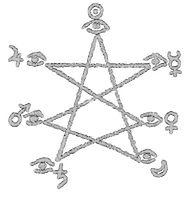The 12 most often appears in the forms 12 (1 x 12), 24 (2 x 12), 36 (3 x 12), 144 (12 x 12) and 216 (18 x 12) or in powers of 10 to 1000.
We live in 3 dimensions and orient ourselves in 4 Cardinal points. (3 x 4 = 12 und 3 + 4 = 7)
Auch im griechischen Olymp wohnten 12 Götter - und 12 Götter gab es auch schon im alten Ägypten.
The 7 appears as a regularly recurring value and stands for perfection: in seven days the world was created and we have the week with 7 days. There are 7 deadly sins and there is talk of "sevens". Jesus had his "Seven Last Sayings". The church modes consist of 7 notes (out of 12) that underlie the 7 modes.
At the time of writing (about 1650 to 2200 years ago), seven planets in our solar system were known except Earth. Saturn has 7 moons. There are 7 chakras and the rainbow (the color spectrum) consists of 7 colors. We have 7 (main) glands.

7 months and (150-) 153 days make a year.

The 666 also appears regularly (666 cases in which the values of the algorithm become larger, or as the sum of the addition of the squared primes up to 17). 666 is the 36th triangular number. The 36th chapter of the Book of Numbers is the 153rd chapter of the Bible.

## Aspects and Permutations

The 153 divides into two aspects, 15 and 53:
10 + 51 + 32 = 15 and 11 + 52 + 33 = 53

Permutations are displacements of the digits. The 153 offers 6 [1+2+3] possible permutations.

The sum of all permutations is 1998. That's 2x 999 oder 3 x 666.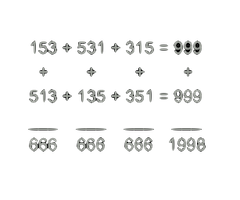153 + 531 + 315 + 513 + 135 + 351 = 1998 = 2 x 999 = 3 x 666

Rotation of the two groups of triple-digits:
(The second row is the first row, from right to left.)

153 + 531 + 315 = 999

513 + 135 + 351 = 999

The added mirrored values (3digits) always result in 666:

153 + 513 = 666

531 + 135 = 666

315 + 351 = 666

Because of the many 666s that appear in the rotation at 153, I consider viewing the "magic" squares as enlightening - and recall that 153 is divisible by both 3 (153 ÷ 3 = 51) [351 is 153 backwards] and 9 (153 ÷ 9 = 17). As a first example I take the simplest form, namely the numbers 1, 2 and 3 in rotation (1,2,3 / 2,3,1 / 3,1,2), which, set in 3 rows, give a 9-field. 3 x 3 = 32.

Both, the addition or multiplication of the digits in a row always yields the sum 6 both horizontally and vertically.

The iterative cross sum from adding the sums (6 + 6 + 6 = 18) is equal to the number of fields of the square in both axes.
Cross sum 18 = 1 + 8 = 9 = 3 x 3 = 32

9 is the base of the triangular number 45:
1+2+3+4+5+6+7+8+9 = 45.
The cross sum of 45 is again 9
4 + 5 = 9 = 3 x 3 = 32.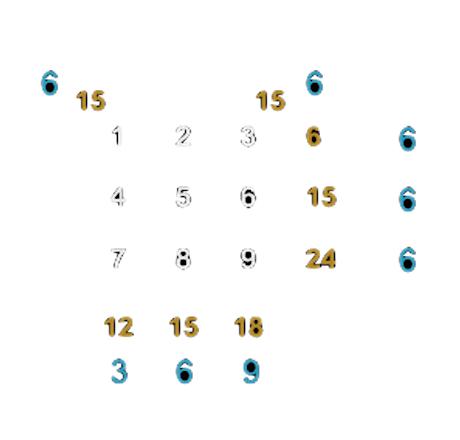The indexing of the fields (1 to 9) produces nothing but sums, divisible by 3 (yellow) whose digit sums (blue) horizontally and diagonally yield 6.

In vertical addition the cross sums (blue) are 3, 6 and 9 - that is 1x3, 2x3 and 3x3. The sums 12, 15 and 18 (yellow) continue and represent 4x3, 5x3 and 6x3.

The unit places of the vertical sums 2, 5, 8, multiplied by 3, result in the same sums as the horizontal addition:
2 x 3 = 6, 5 x 3 = 15, 8 x 3 = 24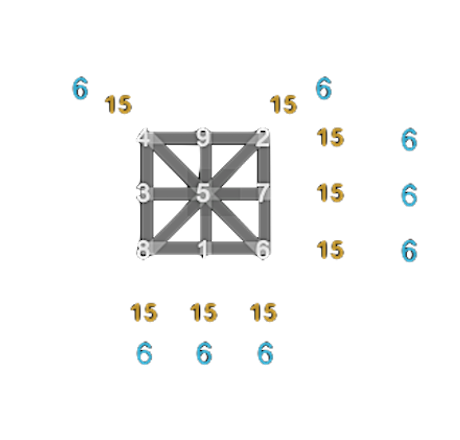By moving the index numbers, all sums, horizontal, vertical and diagonal, can be set to 15, bringing all digit sums to 6. Only the 5 stays in place.

Here we encounter a ΙΧΘΥΣ (ICHTHYS) -like symbol in quadratic form. This exact form can be found both in St. Peter's Square (Vatican) and on the Union Jack, the flag of Great Britain.

## Brief explanation of the Fish Symbol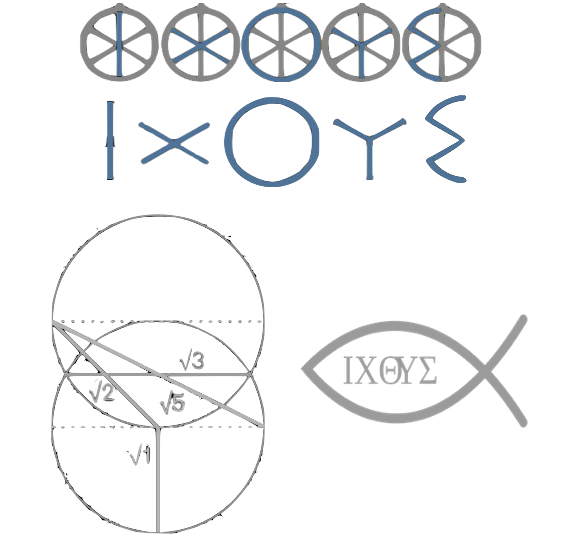ΙΧΘΥΣ (ICHTHYS) is Greek and means "fish" (Gematria value = 153). The symbol is one of the oldest known (early) Christian symbols. ΙΧΘΥΣ (fish) are the initial letters of the Creed ΙΗΣΟΥΣ (Jesus), ΧΡΙΣΤΟΣ (Christ/the Anointed One), ΘΕΟΥ (God), ΥΙΟΣ (Son), ΣΩΤΗΡ (Savior/Redeemer).

These five letters "ΙΧΘΥΣ" may all be written on the existing lines of the 32 square (top right picture). The "original" of the ΙΧΘΥΣ symbol can be seen on the right (in a 5-fold version with one letter each) and is distinctly different from the 32 square: the 32 square has 4 axes and 8 fields á 45º, the ΙΧΘΥΣ wheel has 3 Axes and 6 fields á 60º.

Another interesting thing about the fish symbol: the shape is obtained from the overlapping part of two circles pushed one above the other, with the outer edge of both circles extending to the middle of the other circle. This geometric entity is called "vesica piscis" (fish bubble).
f the distances are calculated exactly, the √1, √5, √3 (and √2) surprisingly results.
(1 2 3 5 is also the beginning of the Fibonacci Sequence.)

The caudal fin of the fish symbol symbolizes the continuous circles.

## Magic Squares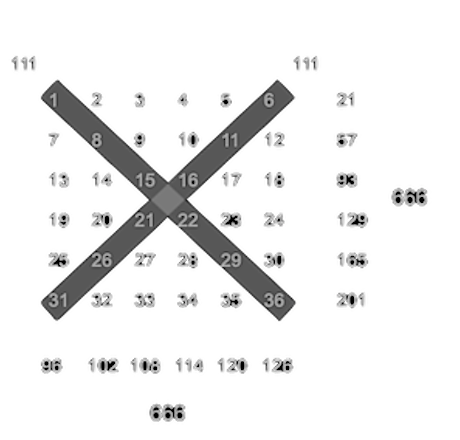The sum 666 is unavoidable in the addition of the 36 fields (6 x 6 = 36 = 62) since 666 is the 36th triangular number.

I find it interesting that, as in the 9 square, all row and column sums are divisible by 3. All horizontal and diagonal iterative digit sums equal 3.

The diagonals make it easy to see that there are 7 steps between the values from top left to bottom right. From top right to bottom left, there are therefore 5 steps because it is in opposite directions. 7+5=12 reminds me of "7 out of 12 tones".

Both diagonals make the sum 111
(1 + 1 + 1 = 3)
111 plays a special role in the 36-field squares and in the Permutation of 153.

For the sum of 666 and 6 rows and columns, 111 (per row/column) is the value at which the "energy" is evenly distributed. This is possible when shifting the index numbers.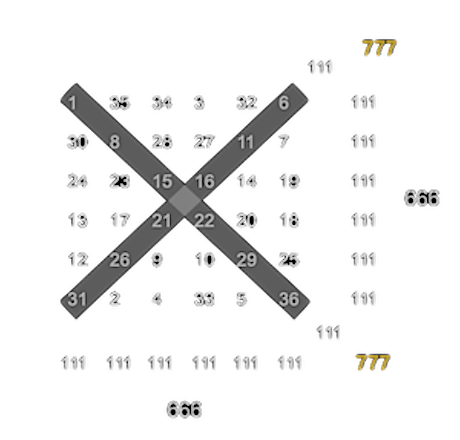All the rows, horizontal, vertical and diagonal, add up to 111. For the sum 666 of the horizontal and vertical rows, there are 6 chances, but the diagonals give 111 only once.

At this position, the diagonal 111 adds the rows to 777. It "surpasses" the Beast with the number 666 (iterated cross sum 9 = finiteness, the "last" of the digits). The 777 (iterated digit sum 3) in numerology stands for perfection.

I find interesting and striking, that both diagonal axes maintain the original index values and the axes are arranged in progressive 5 or 7 intervals (5 + 7 = 12 and 3 x 12 = 36).

Only 24 (2 + 4 = 6) of the 36 index values are changed for this position. These are the 4 x 6 numbers in the four black triangles (4 x 3 = 12). 33.3% of the indices remain in the original positions - and 66.6% needs to be changed.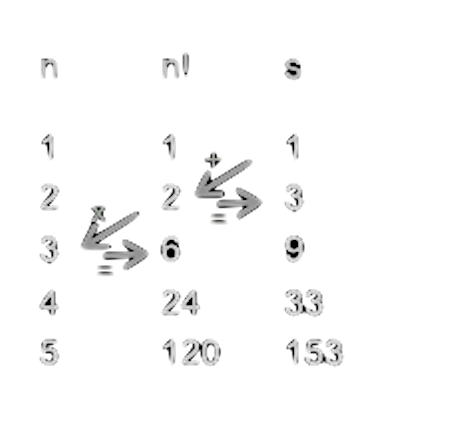Five indexes to the 153:

A form of column encryption - it looks like a standard sewing stitch.
Column n = Index
Column n! = Factorial
Column s = Sum

Encodings that are not (immediately) recognized are more likely to be performed in interleaves - these may be column jumps and changing measure units or language references (e.g., Hebrew, Greek, Latin). Finally, there are enough starting points.

I stick to the simple proportions. It is more a collecting than an explanation. I just want to raise awareness of these numbers and encourage them to perceive the "open" appearance of this fantastic mathematical world.

"But you have set all things in right order by proportion: by measure, by number, and by weight."(three qualities)

Wisdom of Solomon 11:20

## 111 and the 153-Permutations/Rotations

The rotation of 153 (531, 315) yields the following:

153 = 9 x 17 or 32 x 17
531 = 9 x 59 or 32 x 59
315 = 9 x 35 or 32 x 35

Since 35 is not a prime number, but can be represented in primes, we get:
315 = (3x3) x 5 x 7.

Cross sum of (3x3) + 5 + 7 = 21 = 3 x 7. The iterative cross sum is 3.

The Tens digits (of 17, 59, 35) are 1, 5, 3.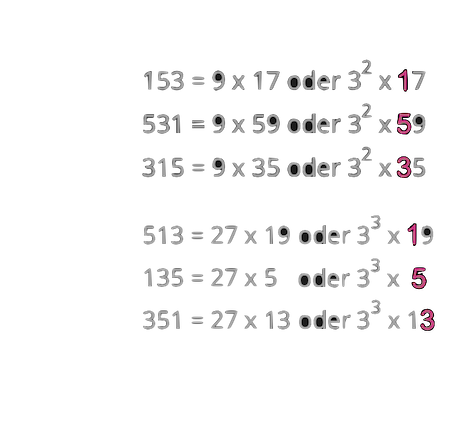The addition of coefficients: 17 + 59 + 35 = 111

Because it is somehow nice and maybe still might open a door: 111 (binary) = 7 (decimal).

The rotation of the remaining 3 permutations
(153, 531 and 315 read from back to front):

513 = 27 x 19 or 33 x 19
135 = 27 x 5 or 33 x 5
351 = 27 x 13 or 33 x 13

Here, too, the result is 153 - but the 1 is a tens digit and 5 and 3 are ones.

It is remarkable that all coefficients in both groups are prime numbers or are to be decomposed into prime numbers.

The Addition of the coefficients 19 + 5 + 13 = 37

And: 3 x 37 = 111

## The TetrakysThe 3 x 37 (= 111) draws the eye to the Tetraktys (the tetrad), which I initially called a compass. After reading this far, only the name (tetrad) and the form (triangle) might produce a certain resonance. It is called (Tetrad =) quaternity because 1 + 2 + 3 + 4 = 10 and the triangle consists of 10 points (i.e., 10 is the fourth triangular number)

The Tetractys (the underlying triangle of 9 triangles) consists of 37 elements:
10 points + 18 lines + 9 surfaces = 37 elements.

1 + 2 + 3 + 4 also means:
point = Dimension 0; line = Dimension 1; surface = Dimension 2; solid figure = Dimension 3

Considering the Hexagon as superimposed, we get 17 points;
Triangle = 10 points + Hexagon = 7 points.
153 is the 17th triangular number.

The inner circle stands to the outer circle in a surface ratio of 1: 3 (or 33,3%).

By connecting the points with lines, the Tetractys opens up 3 dimensions:
Derived are the polyhedra (Platonic solids) - easy to recognize are the hexahedra (cubes/6 faces).

A hexagon (6-sided figure) also corresponds to the two-dimensional representation of a cube (=3). The cube has 6 faces, each of which also covers 360 degrees (6 x 360 = 2160) and stands for the cult worship of Saturn. The Christian cross is also a (2-dimensional) representation of a cube. A craft sheet for a dice would have the shape of a cross.

6 x 360 = 6 x 6 x 60 = 2160 (The moon has a diameter of 2160 miles.)

The full name of God (Old Testament) "Ha-Shem Ha-Mephorash" has 216 letters.

6 x 6 x 6 = 216

At this point, it should be enough with the calculation for now. It could be written infinitely much more and the calculations could be extremely more complex - so far, it's only the surface of the surface. Also, I leave the interpretation completely undiscussed, since I am first of all to show the proportions in our everyday lives. The fact that these ratios are not based on coincidence, should, however, have become clear up to here. It is fascinatingly complex! And yet we move "safely" in this system.

## Where we meet these numbers and relationships in everyday life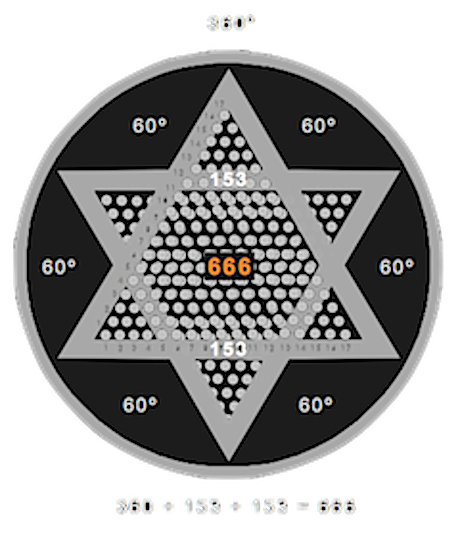In fact, we meet these valences and relationships much more often than we think. Mention may be again the natural sound series, the 7 days, the 7 diatonic tones, the 12 chromatic tones, 12 apostles, 12 months, the day with 2 x 12 hours, 2 x 12 tones (thirds over two octaves) for the church scales and we also have 2 x 12 ribs. Of the 12 ribs, 7 are called "real ribs". The division of the Chronos: 60 seconds = 1 minute, 60 minutes = 1 hour and a day consists of 24 hours (2 + 4 = 6). Here we encounter a covert 666 (zeros are meaningless).

The year did not always have 365 (or 366) days. The "biblical year" lasted 360 days. Here we are reminded of the 360 degrees of the circle. Without zero it is the 36 (triangle number 666). Even the Star of David - two triangles, one of which is upside down, make 6 x 60 degrees. A picture of one of the oldest "Star of David" from Sumer (about 6,000 years) - which, of course, was not so named, amazed me, because the two triangles consisted of many triangles - each with 17 pieces in the base - so every triangle existed from 153 triangles. In the picture on the right I tried to play it in a simplified way.

Especially armorials transport numbers in abundance. Especially logos are often "enriched" by a 666 (see also CERN or Google) - of course, so that it is not immediately recognizable (upside down).

## The ISO formats and Daniel's 70th week

Everything started for me when I noticed the consistency of the DIN (now ISO) formats.
The format A0 is 1189mm high and the Bible has 1189 chapters.
Interesting and noteworthy here is that the Bible is written in 2x 594 and one chapter (= 1189).

An example with the "printer paper" A4:

It is 297mm high and 210mm wide. 297 is half of 594 and the sum of 153 + 144 (12 x 12). 210 is half of 420. 420 refers (without 0) to the 42 months - as during the Tribulation and the Great Tribulation of the End Times (Daniel's 70th Week). In fact, 42 months correspond to 3 x 420 days. A mention of an overlap of 30 days is in Daniel 12:11 "And from the time when the daily sacrifice is abolished and the abomination of desolation is set up, it is 1290 days". It's probably a nesting and both values have their position. It is interesting, however, that the "sevens" describe biblical years. But if 36 (triangle number 666) is added, we will get exact times.

The following presentation should illustrate my assumption - with the help of the A4 ISO format. I have depicted Tribulation and Great Tribulation, each with 1260 days. In addition, it is 2520 days, which is exactly "7 times", i. 84 months (digit sum = 12). The "times" information refers to the biblical year (with 360 days and 12 months with 30 days). If we add the 36 (triangle number 666) to these 2520 days, we get exactly 7 years of our calendar, namely 3 years, 1 leapyear and again 3 years.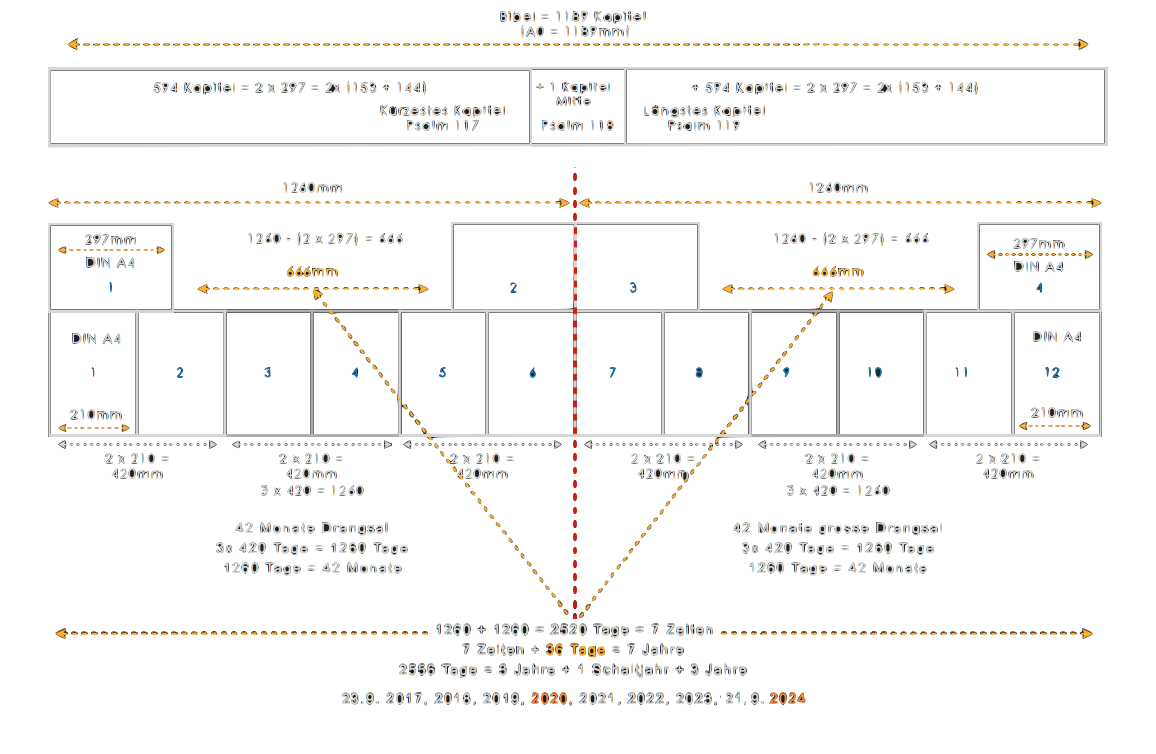This is particularly interesting, because on 9.23.2017 a big date is pending: the beginning of the Apocalypse (Greek: reveal). It repeats a planetary constellation the first time since the birth of the biblical Adam. The date coincides with my A4 tribulation template and meets the leapyear middle position (2017 2018 2019 [2020 leapyear] 2021 2022 2023 [2024 leapyear]) - and not only that: it starts after Yom Teruah (Feast of Trumpets) 2017. So maybe it's not only years, as communicated by the churches, but also days of our time system.